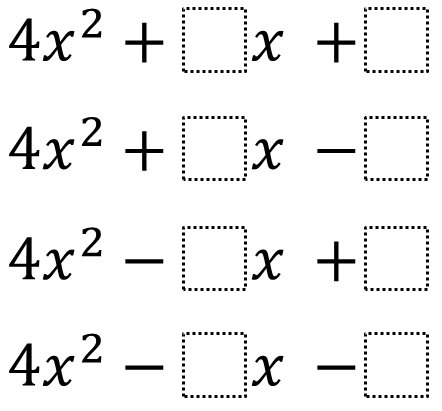Directions: Using the digits 1 to 9 at most one time each, place a digit in each box to construct four different quadratic expressions that can be factored as two binomials with integer coefficients and terms.### Hint

Try starting with a random c (constant term). Use this to determine b (first-degree coefficient).